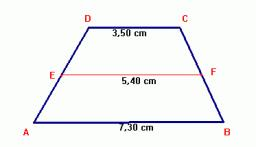# Isosceles 5666

In the isosceles trapezoid ABCD, calculate the missing side length "a" and then its areas. Side b = d = 50 cm, c = 20 cm, height = 48 cm.

a =  48 cm
S =  1632 cm2

### Step-by-step explanation:Did you find an error or inaccuracy? Feel free to write us. Thank you!

Tips for related online calculators
The Pythagorean theorem is the base for the right triangle calculator.